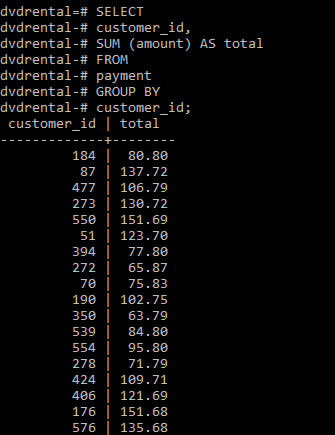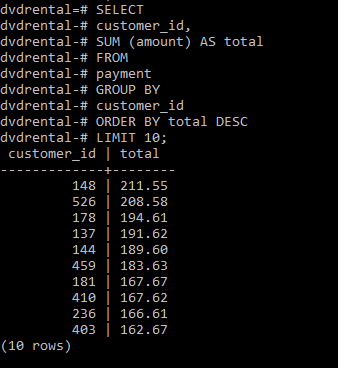PostgreSQL – SUM() Function

• Last Updated : 01 Jun, 2020

PostgreSQL provides with a SUM() function that is used to get the addition of values of a numeric column.

Syntax: SUM(column)

The following points needs to be kept in mind while using the above function:

• It ignores all NULL values.
• If used with DISTINCT operator as SUM(DISTINCT column), it skips duplicate values.
• Using SUM() function with SELECT clause returns NULL instead of Zero.

For examples we will be using the sample database (ie, dvdrental).

Example 1:
In this example we will calculate the total amount paid by each customer using the SUM() function and GROUP BY clause as follows:

SELECT
customer_id,
SUM (amount) AS total
FROM
payment
GROUP BY
customer_id;

Output:Example 2:
In this example we will query for the top 10 customers who paid the most as follows:

SELECT
customer_id,
SUM (amount) AS total
FROM
payment
GROUP BY
customer_id
ORDER BY total DESC
LIMIT 10;

Output:My Personal Notes arrow_drop_up